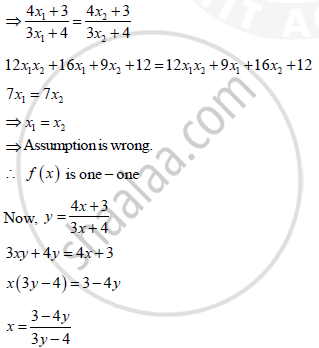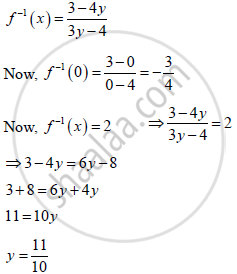# Consider F:R - {-4/3} -> R - {4/3} Given by F(X) = (4x + 3)/(3x + 4). Show that F Is Bijective. Find the Inverse of F and Hence Find F^(-1) (0) And X Such that F^(-1) (X) = 2 - Mathematics

Consider f:R - {-4/3} -> R - {4/3} given by f(x) = (4x + 3)/(3x + 4). Show that f is bijective. Find the inverse of f and hence find f^(-1) (0) and X such that f^(-1) (x) = 2

#### Solution

f(x) = (4x + 3)/(3x + 4)

Assume its not one – one

∴ x1 and x2 belonging to domain such that f(x_1) = f(x_2)`for every ‘y’ there is a x .

∴ it is ontoConcept: Inverse of a Function
Is there an error in this question or solution?